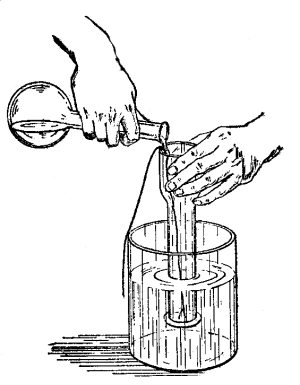## Friday, July 14, 2017

### Equation Money-Energy

Hello Everyone,

I read an interesting explanation for energy, after stating that energy is never depleted as it is the first law of thermodynamics. It explains that in chemistry, this law is demonstrated in the fact that the amount of heat and work are equal to the total energy change in the system.

Also after mentioning that the distribution of heat or work can change, but that they will always equal the same energy change because they are part of a closed system.The explanation is expressed in the equation of:
E (energy) =   H (heat)  +  W (work)

Thus:
Work =  Energy  -  Heat
Heat =  Energy -  Work

Then another example is added, to make it clearer for our minds:

Balance                 =  (Deposited money)  -  (Withdrawn money)